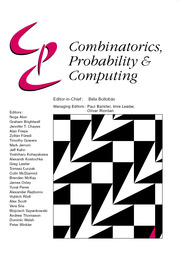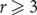Home
Hostname: page-component-558cb97cc8-9njm9 Total loading time: 0.359 Render date: 2022-10-06T05:41:06.408Z Has data issue: true Feature Flags: { "shouldUseShareProductTool": true, "shouldUseHypothesis": true, "isUnsiloEnabled": true, "useRatesEcommerce": false, "displayNetworkTab": true, "displayNetworkMapGraph": true, "useSa": true } hasContentIssue falseCombinatorics, Probability and Computing

# Hamiltonian Berge cycles in random hypergraphs

Published online by Cambridge University Press:  08 September 2020

*
*Corresponding author. Email: patrick.devlin@yale.edu

## Abstract

In this note we study the emergence of Hamiltonian Berge cycles in random r-uniform hypergraphs. For$r\geq 3$ we prove an optimal stopping time result that if edges are sequentially added to an initially empty r-graph, then as soon as the minimum degree is at least 2, the hypergraph with high probability has such a cycle. In particular, this determines the threshold probability for Berge Hamiltonicity of the Erdős–Rényi random r-graph, and we also show that the 2-out random r-graph with high probability has such a cycle. We obtain similar results for weak Berge cycles as well, thus resolving a conjecture of Poole.

## MSC classification

Type
Paper
Information
Combinatorics, Probability and Computing , March 2021 , pp. 228 - 238

## Access options

Get access to the full version of this content by using one of the access options below. (Log in options will check for institutional or personal access. Content may require purchase if you do not have access.)

## Footnotes

An earlier arXiv draft of this paper did not have our stopping time results.

## References

Ajtai, M., Komlós, J. and Szemerédi, E. (1985) First occurrence of Hamilton cycles in random graphs. In Cycles in Graphs (Burnaby, BC, 1982), Vol. 115 of North-Holland Mathematics Studies, pp. 173178. North-Holland.Google Scholar
Alon, Y. and Krivelevich, M. (2020) Random graph’s Hamiltonicity is strongly tied to its minimum degree. Electron. J. Combin. 27 P1.30.CrossRefGoogle Scholar
Berge, C. (1970) Graphes et Hypergraphes, Vol. 37 of Monographies Universitaires de Mathématiques. Dunod.Google Scholar
Bohman, T. and Frieze, A. (2009) Hamilton cycles in 3-out. Random Struct. Algorithms 35 393417.CrossRefGoogle Scholar
Bollobás, B. (1984) The evolution of sparse graphs. In Graph Theory and Combinatorics (Cambridge, 1983), pp. 3557. Academic Press.Google Scholar
Bollobás, B. (2001) Random Graphs, second edition, Vol. 73 of Cambridge Studies in Advanced Mathematics. Cambridge University Press.CrossRefGoogle Scholar
Clemens, D., Ehrenmüller, J. and Person, Y. (2016) A Dirac-type theorem for Hamilton Berge cycles in random hypergraphs. Electron. Notes Discrete Math. 54 181186.CrossRefGoogle Scholar
Cooper, C. and Frieze, A. (2000) Hamilton cycles in random graphs and directed graphs. Random Struct. Algorithms 16 369401.3.0.CO;2-J>CrossRefGoogle Scholar
Devlin, P. and Kahn, J. (2017) Perfect fractional matchings in k-out hypergraphs. Electron. J. Combin. 24 360.CrossRefGoogle Scholar
Dudek, A. and Frieze, A. (2011) Loose Hamilton cycles in random uniform hypergraphs. Electron. J. Combin. 18 P48.CrossRefGoogle Scholar
Dudek, A. and Frieze, A. (2013) Tight Hamilton cycles in random uniform hypergraphs. Random Struct. Algorithms 42 374385.CrossRefGoogle Scholar
Dudek, A. and Helenius, L. (2018) On offset Hamilton cycles in random hypergraphs. Discrete Appl. Math. 238 7785.CrossRefGoogle Scholar
Dudek, A., Frieze, A., Loh, P.-S. and Speiss, S. (2012) Optimal divisibility conditions for loose Hamilton cycles in random hypergraphs. Electron. J. Combin. 19 P44.CrossRefGoogle Scholar
Fenner, T. I. and Frieze, A. M. (1982) On the connectivity of random m-orientable graphs and digraphs. Combinatorica 2 347359.CrossRefGoogle Scholar
Frieze, A. and Karoński, M. (2016) Introduction to Random Graphs. Cambridge University Press.CrossRefGoogle Scholar
Komlós, J. and Szemerédi, E. (1983) Limit distribution for the existence of Hamiltonian cycles in a random graph. Discrete Math. 43 5563.CrossRefGoogle Scholar
Krivelevich, M. (2016) Long paths and Hamiltonicity in random graphs. In Random Graphs, Geometry and Asymptotic Structure (Krivelevich, M. et al., eds), Vol. 84 of London Mathematical Society Student Texts, pp. 427. Cambridge University Press.CrossRefGoogle Scholar
Kühn, D. and Osthus, D. (2014) Hamilton cycles in graphs and hypergraphs: an extremal perspective. Proceedings of the International Congress of Mathematicians—Seoul 2014. Vol. IV, 381–406, Kyung Moon Sa, Seoul.Google Scholar
Parczyk, O. and Person, Y. (2016) Spanning structures and universality in sparse hypergraphs. Random Struct. Algorithms 49 819844.CrossRefGoogle Scholar
Poole, D. (2014) On weak Hamiltonicity of a random hypergraph. arXiv:1410.7446Google Scholar

# Save article to Kindle

To save this article to your Kindle, first ensure coreplatform@cambridge.org is added to your Approved Personal Document E-mail List under your Personal Document Settings on the Manage Your Content and Devices page of your Amazon account. Then enter the ‘name’ part of your Kindle email address below. Find out more about saving to your Kindle.

Note you can select to save to either the @free.kindle.com or @kindle.com variations. ‘@free.kindle.com’ emails are free but can only be saved to your device when it is connected to wi-fi. ‘@kindle.com’ emails can be delivered even when you are not connected to wi-fi, but note that service fees apply.

Find out more about the Kindle Personal Document Service.

Hamiltonian Berge cycles in random hypergraphs
Available formats
×

# Save article to Dropbox

To save this article to your Dropbox account, please select one or more formats and confirm that you agree to abide by our usage policies. If this is the first time you used this feature, you will be asked to authorise Cambridge Core to connect with your Dropbox account. Find out more about saving content to Dropbox.

Hamiltonian Berge cycles in random hypergraphs
Available formats
×

# Save article to Google Drive

To save this article to your Google Drive account, please select one or more formats and confirm that you agree to abide by our usage policies. If this is the first time you used this feature, you will be asked to authorise Cambridge Core to connect with your Google Drive account. Find out more about saving content to Google Drive.

Hamiltonian Berge cycles in random hypergraphs
Available formats
×
×